# Range-Time Parameter Plots

Range-time parameter plots (also known as range-time intensity (RTI) plots) are time series of a radar-measured parameter at all range-gates along a specific beam. They are the most common way to look at data from a single radar.

### Basic RTP

The general syntax for plot_range_time is: 'plot_range_time(fitacf_data, options)' where 'fitacf_data' is the read in data, and the options are several python parameters used to control how the plot looks.

First, make sure pyDARN and matplotlib are imported, then read in the .fitacf file with the data you wish to plot:

``````import matplotlib.pyplot as plt

import pydarn

fitacf_file = "20190831.C0.cly.fitacf"

``````

You can choose one of four data products to plot:

Data product String name
Line of sight velocity (m/s) `v`
Spectral width (m/s) `w_l`
Elevation angle (degrees) `elv`
Power (dB) `p_l`

`parameter=String name`

as an option. The default if left blank is `v`.

To specify which beam to look at, add the option:

`beam=beam_number`

As an example, taking a look at some `v` data from the first record of Clyde River radar FITACF file:

``````pydarn.RTP.plot_range_time(fitacf_data, beam_num=fitacf_data['bmnum'], range_estimation=pydarn.RangeEstimation.RANGE_GATE)

plt.show()
``````

which produces:`fitacf_data['bmnum']` is used to extract the beam number of the first (0th) record from the data dictionary, whilst `fitacf_data['stid']` gives the station id (which is 66 for Clyde River).

Notice that the velocity scale on the right is a bit larger than we need, and also ground scatter isn't coloured grey by default. Showing the dates on the x axis is also a bit redundant, because it's data from a single day. Below, there are some additional parameters you can set to address these and more.

In addition, we use `range_estimation=pydarn.RangeEstimation.RANGE_GATE` to set the y-axis to plot in range gates. Ground-Scatter Mapped Range is another type of axis you can use with range-time plots:

``````pydarn.RTP.plot_range_time(fitacf_data, beam_num=3, parameter='p_l',
range_estimation=pydarn.RangeEstimation.GSMR,
colorbar_label='SNR')
plt.title("Clyde 20150308 14:00 UTC Fitacf 2.5")
plt.ylabel('Ground Scatter Mapped (km)')
plt.xlabel('Time (UTC)')
plt.show()
``````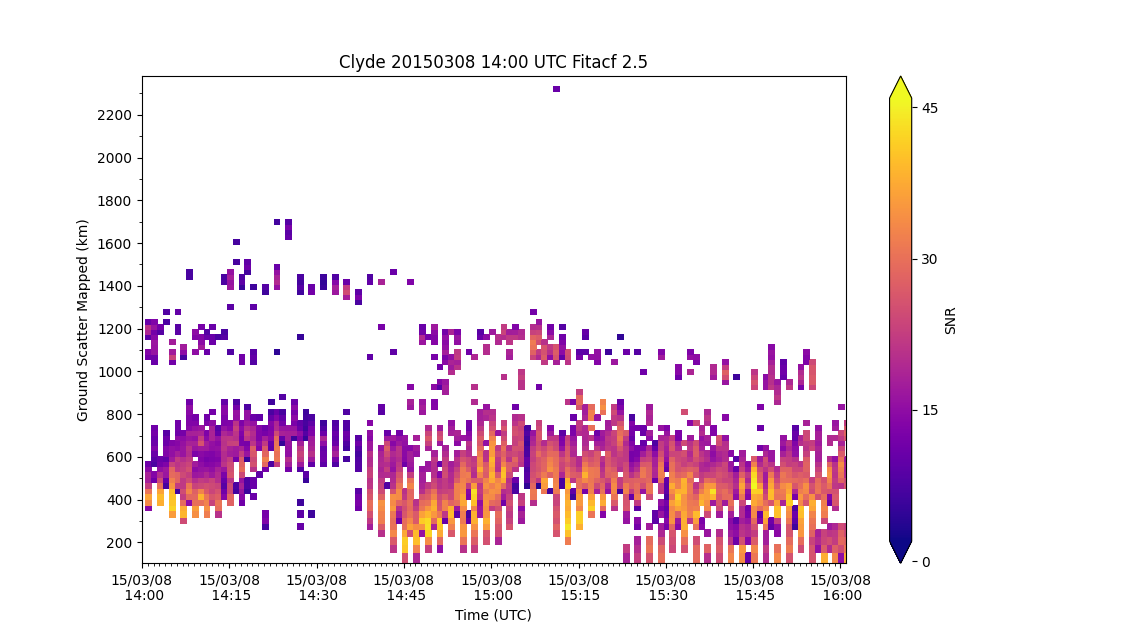To see all the customisation options, check out all the parameters listed in 'rtp.py'. A few useful ones:

Parameter Action
start_time=(datetime object) Control the start time of the plot
end_time=(datetime object) Control the end time of the plot
channel=(int or string) Choose which channel to plot. Default is 'all'.
groundscatter=(bool) True or false to showing ground scatter as grey
date_fmt=(string) How the x-tick labels look. Default is ('%y/%m/%d\n %H:%M')
zmin=(int) Minimum data value to be plotted
zmax=(int) Maximum data value to be plotted
range_estimation=(RangeEstimation) Coordinates to use for the y-axis (See Coordinates)

For instance, code for a velocity RTP showing the same beam of Clyde river radar as above, but with ground scatter plotted in grey, date format as `hh:mm`, custom min and max values and a colour bar label could look something like:

``````pydarn.RTP.plot_range_time(fitacf_data, beam_num=fitacf_data['bmnum'], groundscatter=True,
zmax=500, zmin=-500, date_fmt='%H:%M',
colorbar_label='Line-of-Sight Velocity (m s\$^{-1}\$)',
range_estimation=pydarn.RangeEstimation.RANGE_GATE)
``````

which outputs: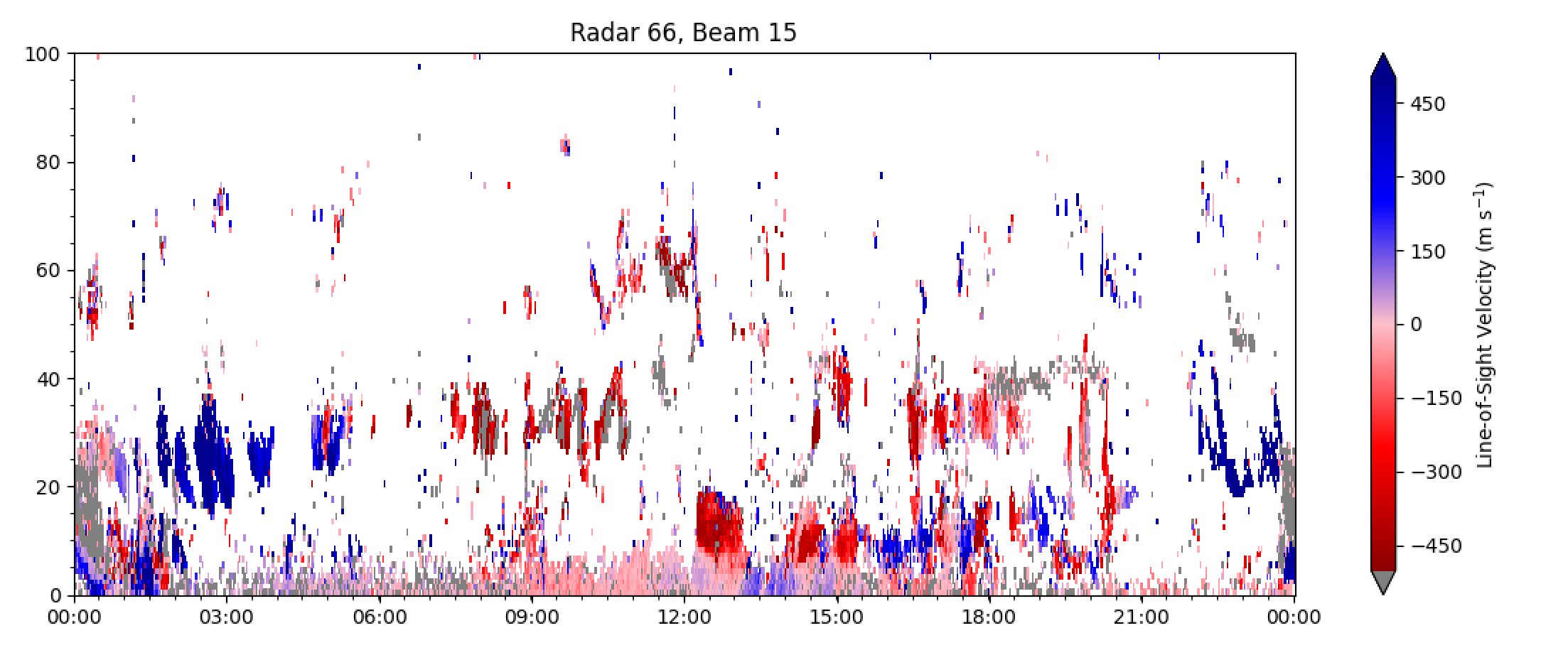and looks much more useful!

#### Plotting with a custom color map

Because the default parameter plotted is line-of-sight velocity, there is also a special red-blue colour map set as default (as seen above) which is only meant for velocity RTP's.

To change the colormap, use the 'cmap' parameter with the string name of a matplotlib color map (found here). For example, plotting the power along the beam above using the colormap 'viridis':

``````pydarn.RTP.plot_range_time(fitacf_data, beam_num=7, parameter='p_l', zmax=50, zmin=0, date_fmt='%H%M', colorbar_label='Power (dB)', range_estimation=pydarn.RangeEstimation.RANGE_GATE, cmap='viridis')
``````

produces: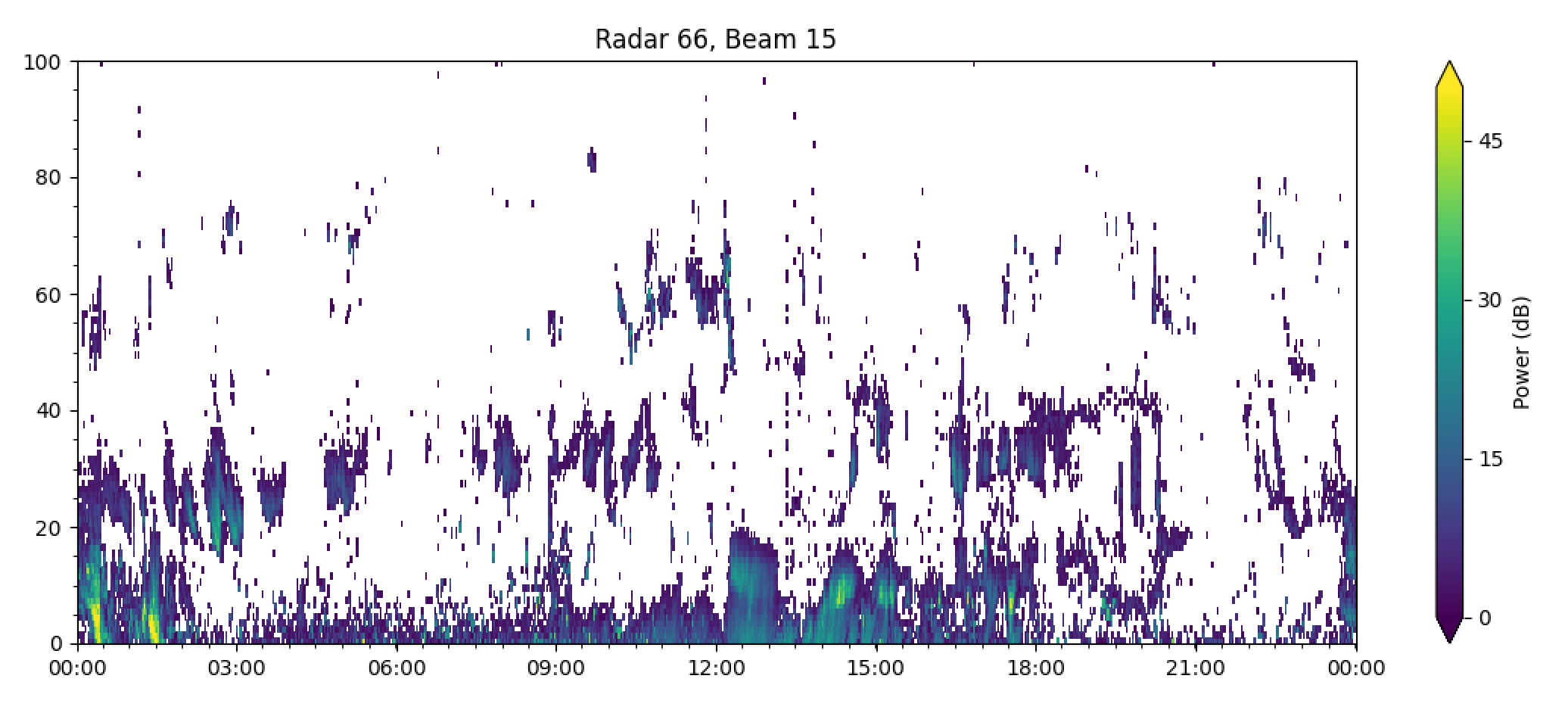Feel free to choose a color map which is palatable for your needs.

Warning

If the data contains `-inf` or `inf` a warning will be presented and the following parameters will be defaults to the scale:

Parameter Name Scale
`v` velocity (-200, 200)
`p_l` Signal to Noise (0, 45)
`w_l` Spectral Width (0, 250)
`elv` Elevation (0, 45)

Example:

``````import pydarn
import pydarnio
import matplotlib.pyplot as plt

fitacf_file = 'data/20161014.1401.00.rkn.lmfit2'
pydarn.RTP.plot_range_time(fitacf_data, beam_num=7, parameter='p_l')
plt.show()
``````

console output

``````UserWarning: Warning: zmin is -inf, set zmin to 0. You canset zmin and zmax in the functions options
``````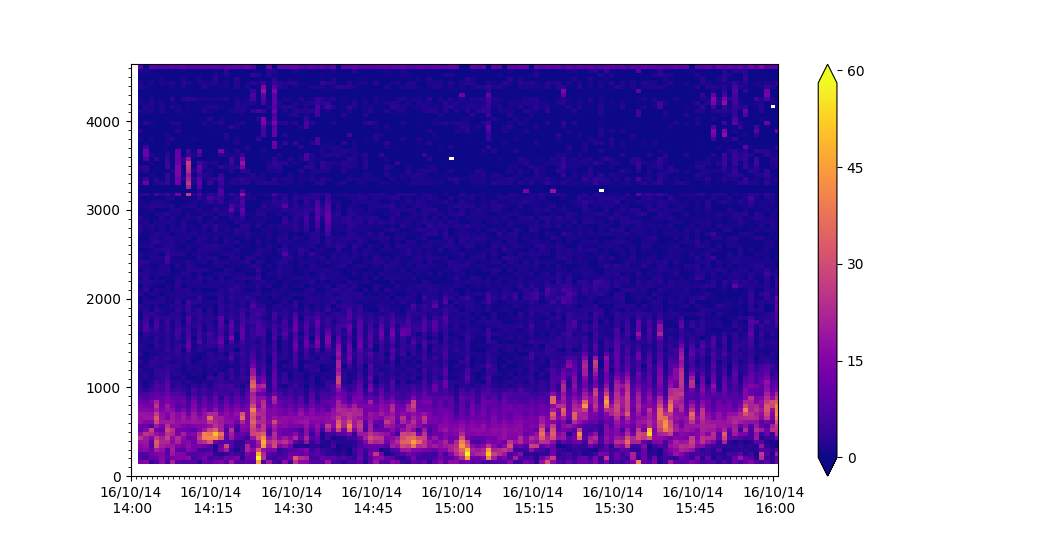Warning

When using filters on data you may remove all data or some data which causes a `NoDataError` or stripping in the plot

Example of data looking stripping from filtering setting

``````filts = {'min_scalar_filter':{'tfreq': 11000}}
pydarn.RTP.plot_range_time(fitacf_data, beam_num=7, parameter='p_l',
filter_settings=filts)
plt.show()
``````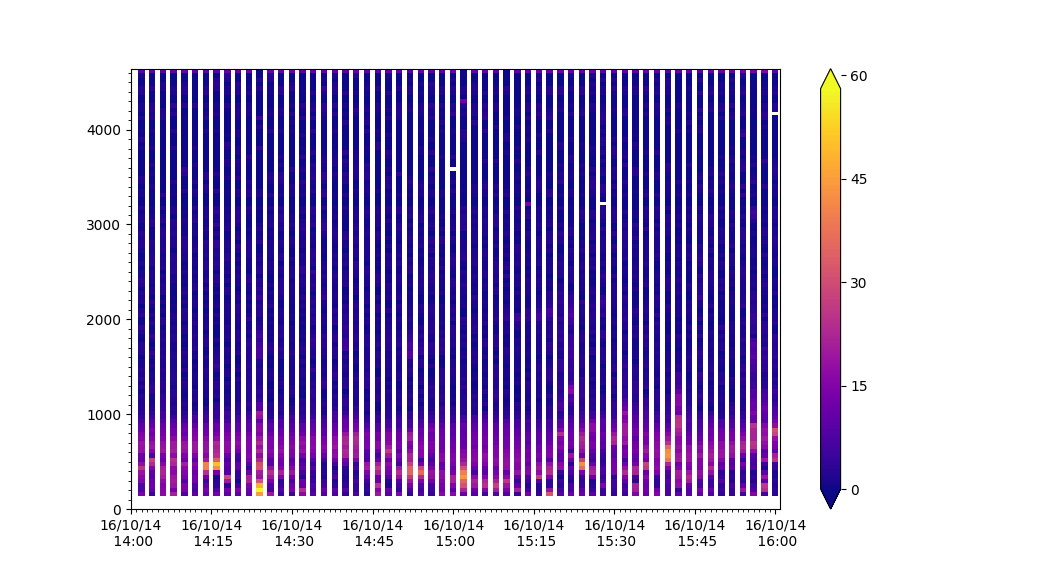### Plotting Lag-0

Range-time plots also allow users to plot `pwr0` parameters in RAWACF files:

``````import pydarn
import matplotlib.pyplot as plt
from matplotlib import colors

rawacf_file = './data/20180404.0601.00.inv.rawacf'